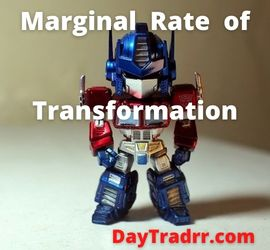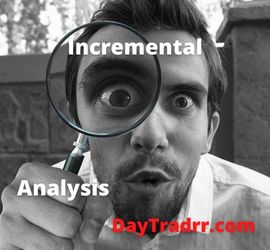# Marginal Rate of Transformation: Formula – Explanation – Example

## What Is the Marginal Rate of Transformation (MRT)?Marginal Rate of Transformation measures opportunity cost.  It shows how many units of one product you need to curtail to make one unit of another.  In other words, MRT deals primarily with economic priorities given limited available resources.

The marginal rate of transformation (MRT) is the number of units or quantity of one good that must be sacrificed in order to generate one unit of another item. It is the number of units of good B that will be foregone in order to create an additional unit of good C.  It further assumes the production factors and technology will be maintained and remain unchanged. The MRT may be calculated for a range of different items. However, the rates will vary based on the goods being compared. For example, Item B’s MRT with regard to Item C is generally different from B’s MRT with respect to Item D.

### Marginal Rate of Transformation and the Production Possibility Frontier

Economists use the marginal rate of transformation (MRT) to calculate the costs of producing an additional unit of a commodity. In turn, this is linked to the Production Possibility Frontier (PPF). The PPF graphically depicts the potential output of two commodities from the same resources. While MRT may be calculated for a variety of items, the rates will vary based on the goods being compared. For example, the MRT of Unit Y will be different from that of Units X and A. When you manufacture more units of one commodity, you will inevitably produce less of the other commodity.  Of course, this assumes you have efficiently reallocated resources on the PPF. As a result, the opportunity cost rises. When more than one item is produced, the potential cost of additional products rises.

The amount by which one output can be increased if another is reduced by a small amount, per unit of the decrease, holding total inputs constant. The marginal rate of transformation can be calculated at the level of the firm, the industry, a country, or the world as a whole. It measures opportunity costs, and is given by the gradient of the production possibility frontier (PPF). (Source: oxfordreference.com)

## Marginal Rate of Transformation Formula

In economics, the marginal rate of transformation is used to represent the supply of goods on the production possibility curve. Using the economics formula below, you can determine (MRT) marginal rate of transformation. Marginal rate of transformation formula:

### MRT = MC (x) / MC (y)

• MC (x) = Marginal cost to produce another unit of Item (x)
• MC (y) = Monetary benefit by cutting production of Item (y)
As a result, the ratio shows you how much Y you must give up in order to generate another X. The marginal rate of transformation (MRT) is computed by dividing the marginal cost of manufacturing another unit of an item by the resources saved by not producing another unit of Y. In other words, the MRT is the marginal cost of production for good X divided by the marginal cost of production for good Y.  It is a ratio of total money for production of one extra unit goods of Item X and the rate of production increased by reducing Item Y production.

## Marginal Rate of Transformation (MRT) – What it Tells You

Economists can use the marginal rate of transformation (MRT) to calculate the opportunity costs of producing one more unit of anything. The potential cost is represented by the loss of production of another specified good. The marginal rate of transformation is related to the production possibility frontier (PPF), which depicts the output potential for two items produced with the same resources. The absolute value of the slope of the production possibility border is denoted by MRT. There is a distinct marginal rate of transformation for each location on the frontier, which is shown as a curved line. This rate is determined by the cost of producing the two items.

Producing more of one thing entails producing less of the other.  This is because resources are allocated efficiently at places along the production potential frontier. In other words, resources utilized to produce one good are diverted from other commodities.  As a result, less other goods can be produced. The marginal rate of transformation is used to quantify this tradeoff. In general, as one progresses along the PPF, the opportunity cost grows as does the absolute value of the MRT. Conversely, the opportunity cost (in units) of the other good rises when more of one product is produced. This is analogous to the law of diminishing returns.

### Why it Matters

The basic idea is that in order to produce anything given available resources, something else must be sacrificed. MRT is the rate at which the other good, which is not produced, costs not to manufacture. In general, organizations aim to achieve an equilibrium where the MRT is near to zero.  However, during times of uncertainty, the MRT can add up to actual cash and significant value. As a result, the MRT addresses what the business foregoes when it chooses to produce x rather than y.

## Example of How to Use the Marginal Rate of Transformation (MRT)

The MRT is the rate at which a small amount of Y can be foregone for a small amount of X. The rate is the opportunity cost of a unit of each good in terms of another. As the number of units of X relative to Y changes, the rate of transformation may also change. For perfect substitute goods, the MRT will equal one and remain constant.

As an example, consider a bakery that produces cakes and cupcakes.  If baking one less cake frees up enough resources to bake 6 more cupcakes, the rate of transformation is 6 to 1 at the margin. Conversely, it costs \$6 to make a cake. Meanwhile, \$1 can be saved by not making a cupcake. Thus, the MRT is 6, or \$6 divided by \$1.

## Marginal Rate of Transformation vs Marginal Rate of Substitution

Marginal rate of transformation (MRT) and the marginal rate of substitution (MRS) are comparable.  However, they are not the same. The marginal rate of transformation (MRT) is concerned with supply.  Conversely, the marginal rate of substitution (MRS) is concerned with demand. The marginal rate of substitution indicates how many units of Y a particular consumer group would perceive to be compensation for one less unit of X. For example, consider a customer who likes pears over peaches.  Comparable satisfaction occurs only if she receive three peaches instead of one pear.

As a limitation, the marginal rate of transformation (MRT) is generally not constant.  As a result, it may need to be recalculated frequently. Furthermore, goods will not be distributed efficiently if MRT doesn’t equal MRS.

### What is Marginal Cost vs MRT?

A simpler metric is marginal cost (MC). It is simply the cost of producing more of an item given the fixed quantity of capital at your disposal. If your current capital configuration is only at 50% capacity, your marginal costs for producing new things will be quite cheap. However, it will be quite high if you are already operating at full capacity. This is because both workers and equipment will be stretched to keep up with demand. As a result, MC must cope with both available capital and product demand. Even if your present capital configuration is strained as a result of the increasing demand, the greater demand will push up pricing and compensate for increased production expenses, like overtime.

## Up Next: What Is Incremental Analysis?Incremental analysis is a business decision-making tool that examines alternative choices based on marginal cost differences between them. Incremental analysis is used in business to determine the true cost difference between alternatives.

This technique is also known as the relevant cost method, marginal analysis, or differential analysis.  Simply put, it disregards any sunk or prior costs. Incremental analysis is important in company planning. For example,  deciding whether to self-produce or outsource a function. Companies use this tool to determine whether to accept new business, manufacture, or purchase items.  Also, to sell or process products further, discontinue a product or service, and allocate resources. Only two courses of action are considered and the comparison is based on the differences rather than commonalities.